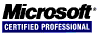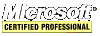## The C++ Copy Constructor

The C++ copy constructor is very important when working with objects. It allows for objects to be passed by value to function arguments and then returned from functions. If a class dynamically allocates memory then it must have a custom copy constructor written. (Also, the assignment operator should be overloaded.) All of the data structures on this site implement copy constructors:

``````#include <iostream>
using namespace std;

class CopyCounter
{
private:
int copyCount;
public:
CopyCounter() : copyCount(0) {}
~CopyCounter() { cout << "destroy copy count " << copyCount << endl; }
CopyCounter(const CopyCounter &obj) : copyCount(obj.copyCount + 1) // custom copy constructor
{
cout << "copy operation under way; copy number " << copyCount << endl;
}
};

CopyCounter MakeCopies(CopyCounter byValue) // invoke copy constructor
{
return byValue; // invoke copy constructor & destroy this copy
}

int main()
{
CopyCounter c0;
CopyCounter c1 = MakeCopies(c0); // this makes a few copies and destroys one copy
CopyCounter c2 = MakeCopies(c1); // this makes a few copies and destroys one copy
CopyCounter c3 = c2; // this just makes one copy (copy 5)
return 0;
}``````Here, the `DynamicMem` class is using dynamic memory and the copy constructor generated by the compiler does not know how to make a copy of it because it does not know the size of the data that `str` points to. Run this and there will be a violation.

``````#include <iostream>
using namespace std;

class DynamicMem
{
private:
char *str;
public:
DynamicMem()
{
cout << "creating *str" << endl;
str = new char; // make str == "abc"
str = 'a';
str = 'b';
str = 'c';
str = 0;
}
~DynamicMem()
{
cout << "deleting *str" << endl;
delete[]str;
}
const char *get() const
{
cout << "getting *str" << endl;
return str;
}
};

DynamicMem MakeCopies(DynamicMem byValue) // invoke copy constructor
{
return byValue; // invoke copy constructor & destroy this copy
}

int main()
{
DynamicMem dm0;
cout << dm0.get() << endl;
DynamicMem dm1 = MakeCopies(dm0);
cout << dm0.get() << endl;
cout << dm1.get() << endl;
return 0;
}
``````

The key is to make a custom copy constructor and not rely on the one provided by the compiler. Run this code. Now it works without a violation.

``````#include <iostream>
using namespace std;

class DynamicMem
{
private:
char *str;
public:
DynamicMem()
{
cout << "creating *str" << endl;
str = new char; // make str == "abc"
str = 'a';
str = 'b';
str = 'c';
str = 0;
}
DynamicMem(const DynamicMem &obj) // CUSTOM COPY CONSTRUCTOR
{
cout << "creating *str in custom copy constructor" << endl;
str = new char; // make str == obj.str
*(int*)str = *(int*)obj.str; // this is a little trick to fit the whole string in a register
~DynamicMem()
{
cout << "deleting *str" << endl;
delete[]str;
}
const char *get() const
{
cout << "getting *str" << endl;
return str;
}
};

DynamicMem MakeCopies(DynamicMem byValue) // invoke copy constructor
{
return byValue; // invoke copy constructor & destroy this copy
}

int main()
{
DynamicMem dm0;
cout << dm0.get() << endl;
DynamicMem dm1 = MakeCopies(dm0);
cout << dm0.get() << endl;
cout << dm1.get() << endl;
return 0;
}
``````Alternatively, the `string` class could have been used here and then there would be no need to write a custom copy constructor. This is because the `string` class has its own custom copy constructor as it uses dynamic memory.

``````#include <string>
#include <iostream>
using namespace std;

class DynamicMem
{
private:
string str;
public:
DynamicMem() : str("abc")
{
cout << "creating str" << endl;
}
const string &get() const // notice that this method returns a reference to the string object "str"
{
cout << "getting str" << endl;
return str;
}
};

DynamicMem MakeCopies(DynamicMem byValue) // invoke copy constructor
{
return byValue; // invoke copy constructor & destroy this copy
}

int main()
{
DynamicMem dm0;
cout << dm0.get() << endl;
DynamicMem dm1 = MakeCopies(dm0);
cout << dm0.get() << endl;
cout << dm1.get() << endl;
return 0;
}
``````

#### "Privatize" the Copy Constructor

Make a copy constructor private to prevent the object from being copied.

``````#include <iostream>
using namespace std;

class CopyCounter
{
private:
int copyCount;
CopyCounter(const CopyCounter &obj) {} // private custom copy constructor
public:
CopyCounter() : copyCount(0) {}
};

CopyCounter MakeCopies(CopyCounter byValue) // invoke copy constructor
{
return byValue; // invoke copy constructor
}

int main()
{
CopyCounter copy;
MakeCopies(copy); // can't do this
CopyCounter copy2 = copy; // can't do this
return 0;
}
``````

### Coding Video

https://youtu.be/jMtMbtbCNq8